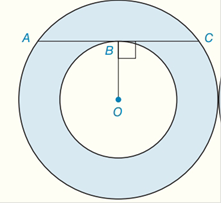Chapter 8.CR, Problem 36CRElementary Geometry For College St...

7th Edition
Alexander + 2 others
ISBN: 9781337614085

Solutions

Chapter
SectionElementary Geometry For College St...

7th Edition
Alexander + 2 others
ISBN: 9781337614085
Textbook Problem

Given : Concentric circles with radii of lengths R and r, with R > r Prove : A r i n g = π ( B C ) 2To determine

To prove:

Aring=π(BC)2

Explanation

Calculation:

Consider the diagram as shown below:

Lengths of the radii of the above concentric circles are R and r with R>r.

Join the line segment OC¯, this is the radius of the outer circle.

We call r the radius of the smaller circle. The radius of the larger circle is:

R=BC2+r2R2=BC2+r2

Area of the shaded region is subtracting the area of the small circle from the area of the larger circle.

Thus, the area of the ring is:

Still sussing out bartleby?

Check out a sample textbook solution.

See a sample solution

The Solution to Your Study Problems

Bartleby provides explanations to thousands of textbook problems written by our experts, many with advanced degrees!

Get Started

Find more solutions based on key concepts